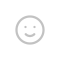Python 中，代码的写法和我 《Python学习日记 – 素数判断》 的有一点出入，因为引入了 time 模块，为程序计时，而 C# 中使用了 System.Diagnostics 对程序进行计时，二者均为语言的内置模块，对程序运行并无太大影响。

Console.Write("请输入一个整数：");
int ConutNumber = 0;
for (int i = 1; i < InputNumber; i++)
{
if (InputNumber % i == 0)
{
ConutNumber = ConutNumber + 1;
}
}
if (ConutNumber >= 2)
{
Console.WriteLine($"{InputNumber} 不是素数"); } else { Console.WriteLine($"{InputNumber} 是素数");
}

x = int(input('输入一个数：'))
z = 0
for i in range(1,x+1):
if x % i == 0:
z = z+1
if z >= 2:
print(f"{x} 不是素数")
else:
print(f"{x} 是素数")

if z >= 2:
break;

## 发送评论编辑评论Emoji